# Overview

When two resonances are close together they may interact. This can distort the amplitude field of the primary resonance and/or may cause the power supply to jump to the secondary (parasitic) resonance.

## Example

The following shows an example of modal interactions for a 20 kHz cylindrical prismatic horn ($$c_{tw}$$ = 5100 m/sec; Poisson's ratio = 0.33). The horn's diamter was adjusted beetween 38 mm and 41 mm in order to affect the amount of modal interaction. The horn's length was adjusted slightly (between 126.54 mm and 126.62 mm) to maintain the axial resonance at 20 kHz. As expected, the modal interaction is greatest when the frequency separation is smallest. (Also see Amplitude asymmetry.)Figure 1. Frequencies for a 20 kHz $$\phi$$40 mm horn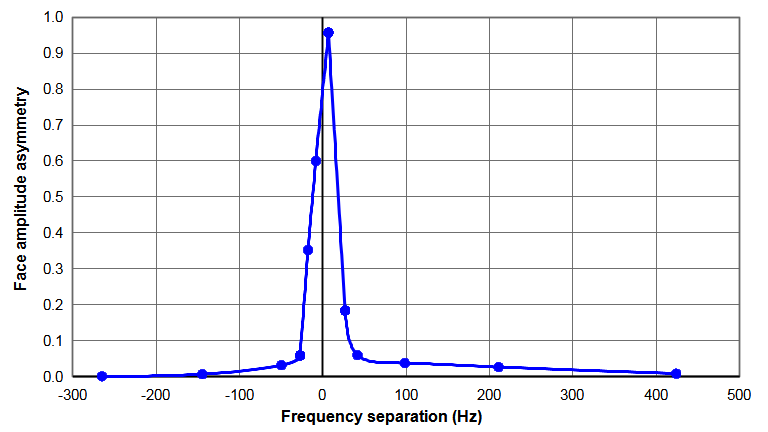Figure 2. Face amplitude asymmetries for a 20 kHz $$\phi$$40 mm horn

The following table shows some data from the above graphs. When the nonaxial (bending) mode is close to the axial resonance (low frequency separation), small changes in the horn's diameter cause large changes in the amplitude asymmetry. In particular, the face motion of the axial resonance starts to assume characteristics of the bending resonance. When the frequency separation is sufficiently large, the axial mode performs as if the bending mode were not present.

Table notes —

1. All displacements (as represented by the image colors) are in the axial direction.
2. All displacements are displayed at the same scale.

Diameter (mm) Frequency
separation (Hz)
Axial mode Nonaxial mode
38.00 -26520005 Hz Asymmetry = 0.00119740 Hz
39.00 -2720000 Hz Asymmetry = 0.06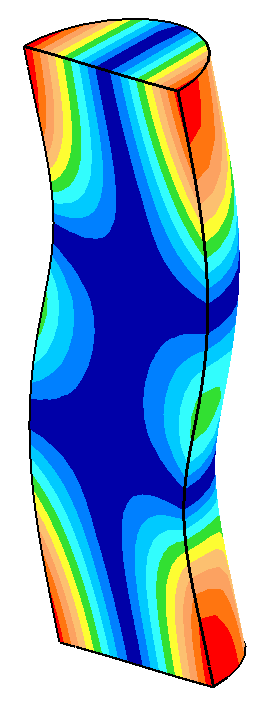19973 Hz
39.02 -1819998 Hz Asymmetry = 0.3419982 Hz
39.05 -1220000 Hz Asymmetry = 0.50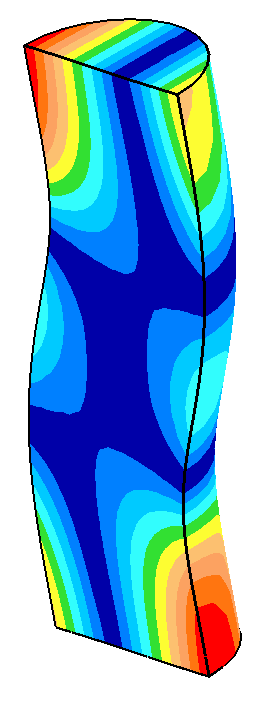19988 Hz
39.10 6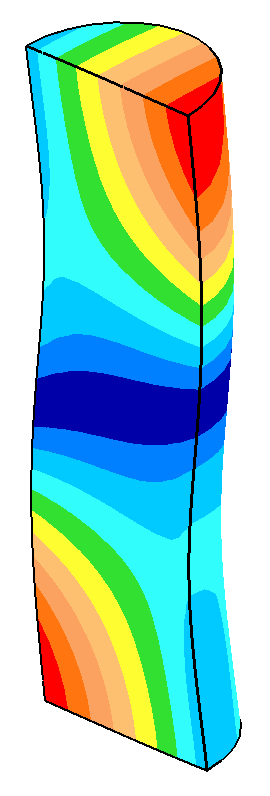20000 Hz Asymmetry = 0.96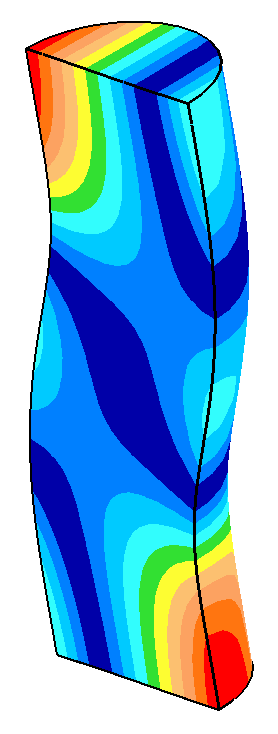20006 Hz
39.20 27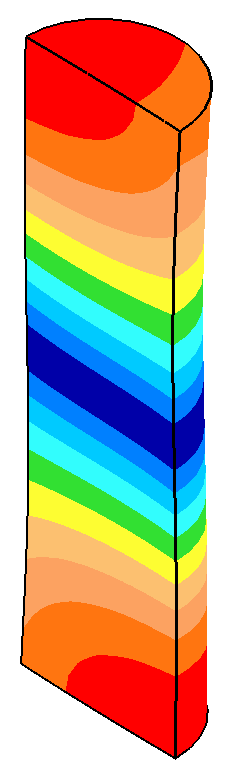20000 Hz Asymmetry = 0.1820027 Hz
39.30 42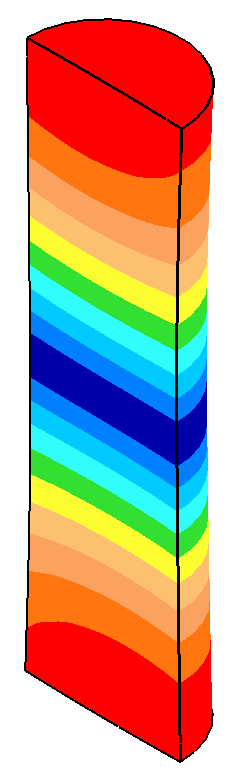20001 Hz Asymmetry = 0.0620043 Hz
41.00 42419996 Hz Asymmetry = 0.008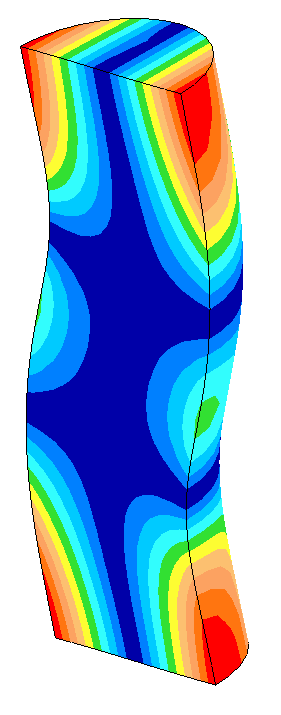20420 Hz

# Suggested frequency separation

In an optimization of a 20 kHz 4.5" x 6" (114 mm x 152 mm) titanium block horn, O'Shea  (p. 260) specified a target frequency separation of at least 1200 Hz between the axial and nonaxial resonances. The reason for this target wasn't specified. If the reason was to avoid frequency jump then it should be noted that power supply controls have become much more sophisticated since this 1991 presentation so a narrower frequency range may be possible. In fact, horns having frequency separations of 500 Hz at 20 kHz have been successful.

Liesegang[1A], p.10 specified a frequency separation of 1000 Hz at 20 kHz.